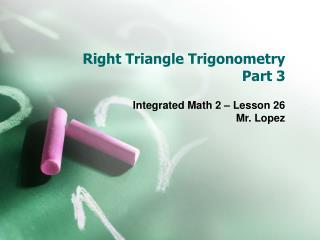Download PresentationRight Triangle Trigonometry Part 3Right Triangle Trigonometry Part 3 - PowerPoint PPT Presentation

Download PresentationRight Triangle Trigonometry Part 3
An Image/Link below is provided (as is) to download presentation

Download Policy: Content on the Website is provided to you AS IS for your information and personal use and may not be sold / licensed / shared on other websites without getting consent from its author. While downloading, if for some reason you are not able to download a presentation, the publisher may have deleted the file from their server.

- - - - - - - - - - - - - - - - - - - - - - - - - - - E N D - - - - - - - - - - - - - - - - - - - - - - - - - - -
Presentation Transcript

1. Right Triangle Trigonometry Part 3 Integrated Math 2 – Lesson 26 Mr. Lopez

2. GO! DO! • In ABC C is 90. B is 60 degrees and BC = 12. Find then length of AB.

3. Review of Right Triangle Trig Ratios • We can determine the sides and the angles of right triangles using trigonometric ratios. • The 3 trig ratios are:

4. S-O-H C-A-H T-O-A • S-O-H C-A-H T-O-A is the acronym for remembering the trig ratios. Sine Cosine Tangent Opposite Adjacent Opposite Hypotenuse Hypotenuse Adjacent

5. Inverse Trig Functions • The inverse trig functions are the functions used to remove a trigonometric sign. • The inverse trig functions are: • sin-1 • cos-1 • tan-1 • We use the inverse trig functions to find a missing angle in right triangle.

6. Practice using the inverse trig functions • Solve for “x” rounded to the nearest degree • sin x = .5876 • cos x = .1254 • tan x = .3679 • sin x = (11/15) • cos x = (2/9) • tan x = (8/5)

7. Using Inverse Trig Function with triangles • In triangle BIG, I is 90. If side IB is 20 and side BG is 30. What is the measure of angle B rounded to the nearest degree?

8. Finding the angle • Step 1: Draw out your diagram and label what sides are opposite, adjacent and hypotenuse all with any information about the problem. B x HYP ADJ 30 20 I G OPP

9. Finding the angle • Step 2: Determine which trig function to use based on the diagram. Remember to draw two arrows from the given angle to the two sides in the triangle containing information. B COS = ADJ/HYP x HYP COS x = 20/30 ADJ 30 20 I G OPP

10. Finding the angle • Step 3: Solve for “x” by the inverse trig functions COS x = 20/30 COS-1(20/30) x  48

11. Try again! • In triangle JOB, O is 90 degrees. If JO is 7 and OB is 15, what is the measure of angle J?